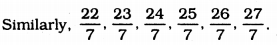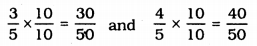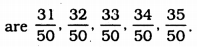# KSEEB Solutions for Class 9 Maths Chapter 1 Number Systems Ex 1.1

In this chapter, we provide KSEEB SSLC Class 9 Maths Chapter 1 Number Systems Ex 1.1 for English medium students, Which will very helpful for every student in their exams. Students can download the latest KSEEB SSLC Class 9 Maths Chapter 1 Number Systems Ex 1.1 pdf, free KSEEB SSLC Class 9 Maths Chapter 1 Number Systems Ex 1.1 pdf download. Now you will get step by step solution to each question.

## Karnataka Board Class 9 Maths Chapter 1 Number Systems Ex 1.1

KSEEB Solutions For Class 9 Maths Question 1.
Is a zero a rational number? Can you write it in the form pq, where p and q are integers and q ≠ 0 ? (p, q,ϵ Z, q ≠ 0)
Zero is a rational number.
This can be written in the form of pq because oq is a rational number.
E.g. 02=0,05=0. etc.
Zero belongs to set of rational number.

KSEEB Solutions For Class 9 Maths Number System Question 2.
Find six rational numbers between 3 and 4.
We can write six rational numbers between 3 and 4 as
3=217 and 4=287
∴ rational numbers between 217 and 287.KSEEB Solutions For Class 9 Maths Chapter 1 Question 3.
Find five rational numbers between 35 and 45
Rational numbers between 35 and 45 are∴ Rational numbers between 3050 and 4050KSEEB Solutions For Class 9 Maths Chapter 1 Number System Question 4.
State whether the following statements are true or false. Give reasons for your answers :
(i) Every natural number is a whole number.
True. Because set of natural numbers belongs to a set of whole numbers.
∴ W = {0, 1, 2, 3 …………………….}

(ii) Every integer is a whole number.
False. Because zero belongs to a set of integers. But -2, -3, -1 are not whole numbers.

(iii) Every rational number is a whole number.
False. Because 12 is a rational number but not a whole number.

All Chapter KSEEB Solutions For Class 9 Maths

—————————————————————————–

All Subject KSEEB Solutions For Class 9

*************************************************

I think you got complete solutions for this chapter. If You have any queries regarding this chapter, please comment on the below section our subject teacher will answer you. We tried our best to give complete solutions so you got good marks in your exam.

If these solutions have helped you, you can also share kseebsolutionsfor.com to your friends.

Best of Luck!!